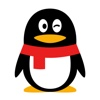/ 猿问

请问关于python django字符串操作?

2019-09-19 14:14:37

python django字符串操作?

5 回答DataFrame类似于numpy中的二维数组，同样可以通用numpy数组的函数和方法，而且还具有其他灵活应用，后续会介绍到。:之前的是app名，即polls app，:之后的是url路由中用name关键字指定的函数名，即name=vote的函数，question.id是传递给该URL的参数。1. 使用连接符： +

world = "World"print "Hello " + world + " ! "

2. 使用占位符来内插

world = "World"print "Hello %s !" % world

3. 使用函数

li = ['my','name','is','bob']mystr = ' '.join(li)print mystr

begin_date = '2012-04-06 00:00:00'end_date = '2012-04-06 23:59:59'select * from usb where time between to_date(begin_date,'YYYY-MM-DD HH24:MI:SS') and to_date(end_date,'YYYY-MM-DD HH24:MI:SS')qq_遁去的一_1#原始数据为2行3列，行标01，列标abc
df = pandas.DataFrame(numpy.random.randn(2, 3), columns=['a', 'b', 'c'])
#增加一列d
df['d'] = pandas.Series(numpy.random.randn(len(df['a'])), index=df.index)
#增加一行2
df.ix = pandas.Series(numpy.random.rand(4),index=df.columns)

• 5 回答
• 0 关注
• 107 浏览

0/150